# Sample Data: Anscombe Regression Lines

Anscombe's 4 regression line data

These four datasets that have nearly identical simple descriptive statistics, yet appear very different when graphed. Each dataset consists of eleven (x,y) points. They were constructed in 1973 by the statistician Francis Anscombe to demonstrate both the importance of graphing data before analyzing it and the effect of outliers on statistical properties.

## Examples

### Basic Examples

#### Retrieve the ResourceObject:

 In:=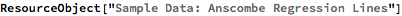Out=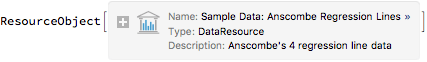#### View the data:

 In:=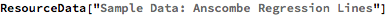Out=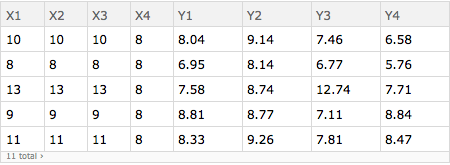### Visualization

#### Plot the four datasets with linear regression line:

 In:=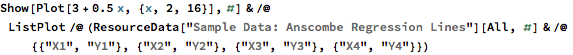Out=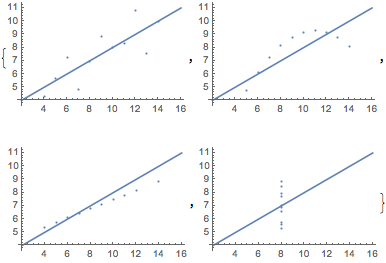Wolfram Research, "Sample Data: Anscombe Regression Lines" from the Wolfram Data Repository (2016) https://doi.org/10.24097/wolfram.70071.data